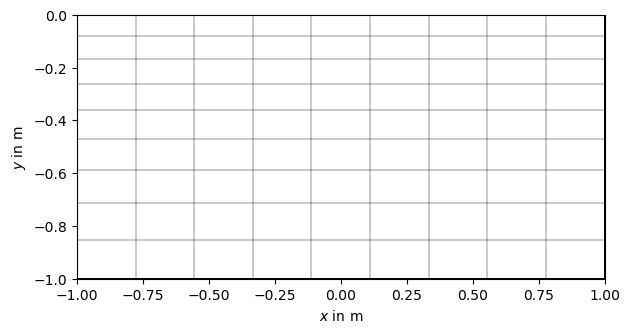# GIMLi basics#

This is the first tutorial where we demonstrate the general use of GIMLi in Python, i.e., pyGIMLi.

The modelling as well as the inversion part of GIMLi often requires a spatial discretization for the domain of interest, the so called GIMLI::Mesh. This tutorial shows some basic aspects of handling a mesh.

First, the library needs to be imported. To avoid name clashes with other libraries we suggest to `import pygimli` and alias it to the simple abbreviation `pg`: CR

```import pygimli as pg
```

Every part of the c++ namespace GIMLI is bound to python and can be used with the leading `pg.`

For instance get the current version for GIMLi with:

```print(pg.__version__)
```
```1.4.5
```

Now that we know the name space GIMLI, we can create a first mesh. A mesh is represented by a collection of nodes, cells and boundaries, i.e., geometrical entities.

Note

A regularly spaced mesh consisting of rectangles or hexahedrons is usually called a grid. However, a grid is just a special variant of a mesh so GIMLi treats it the same. The only difference is how they are created.

GIMLi provides a collection of tools for mesh import, export and generation. A simple grid generation is built-in but we also provide wrappers for unstructured mesh generations, e.g., Triangle, Tetgen and Gmsh. To create a 2d grid you need to give two arrays/lists of sample points in x and y direction, in that order, or just numbers.

```grid = pg.createGrid(x=[-1.0, 0.0, 1.0, 4.0], y=[-1.0, 0.0, 1.0, 4.0])
```

The returned object `grid` is an instance of GIMLI::Mesh and provides various methods for modification and io-operations. General information about the grid can be printed using the simple print() function.

```print(grid)
```
```Mesh: Nodes: 16 Cells: 9 Boundaries: 24
```

Or you can access them manually using different methods:

```print('Mesh: Nodes:', grid.nodeCount(),
'Cells:', grid.cellCount(),
'Boundaries:', grid.boundaryCount())
```
```Mesh: Nodes: 16 Cells: 9 Boundaries: 24
```

You can iterate through all cells of the general type GIMLI::Cell that also provides a lot of methods. Here we list the number of nodes and the node ids per cell:

```for cell in grid.cells():
print("Cell", cell.id(), "has", cell.nodeCount(),
"nodes. Node IDs:", [n.id() for n in cell.nodes()])

print(type(grid.cell(0)))
```
```Cell 0 has 4 nodes. Node IDs: [0, 1, 5, 4]
Cell 1 has 4 nodes. Node IDs: [1, 2, 6, 5]
Cell 2 has 4 nodes. Node IDs: [2, 3, 7, 6]
Cell 3 has 4 nodes. Node IDs: [4, 5, 9, 8]
Cell 4 has 4 nodes. Node IDs: [5, 6, 10, 9]
Cell 5 has 4 nodes. Node IDs: [6, 7, 11, 10]
Cell 6 has 4 nodes. Node IDs: [8, 9, 13, 12]
Cell 7 has 4 nodes. Node IDs: [9, 10, 14, 13]
Cell 8 has 4 nodes. Node IDs: [10, 11, 15, 14]
```

To generate the input arrays `x` and `y`, you can use the built-in GIMLI::Vector (pre-defined with values that are type double as `pg.Vector`), standard python lists or numpy arrays, which are widely compatible with GIMLi vectors.

```import numpy as np

grid = pg.createGrid(x=np.linspace(-1.0, 1.0, 10),
y=1.0 - np.logspace(np.log10(1.0), np.log10(2.0), 10))
```

We can find that this new `grid` contains

```print(grid.cellCount())
```
```81
```

rectangles of type GIMLI::Quadrangle derived from the base type GIMLI::Cell, edges of type GIMLI::Edge, which are boundaries of the general type GIMLI::Boundary.

```print(grid.boundaryCount())
```
```180
```

The mesh can be saved and loaded in our binary mesh format `.bms`. Or exported into `.vtk` format for 2D or 3D visualization using Paraview.

However, we recommend visualizing 2-dimensional content using python scripts that provide better exports to graphics files (e.g., png, pdf, svg). In pygimli we provide some basic post-processing routines using the matplotlib visualization framework. The main visualization call is `pygimli.viewer.show` which is sufficient for most meshes, fields, models and streamline views.

```pg.viewer.show(grid)
pg.wait()
```For more control you can also use the appropriate draw methods `pygimli.viewer.mpl.drawMesh`.

Gallery generated by Sphinx-Gallery